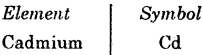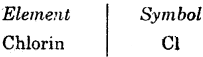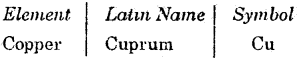# Bihar Board Class 9 Science Solutions Chapter 3 Atoms and Molecules

## BSEB Bihar Board Class 9 Science Solutions Chapter 3 Atoms and Molecules

Bihar Board Class 9 Science Solutions Chapter 3 Atoms and Molecules Textbook Questions and Answers, Additional Important Questions, Notes.

### Bihar Board Class 9 Science Chapter 3 Atoms and Molecules InText Questions and Answers

Intext Questions

Pages 32-33

Question 1.
In a reaction, 5.3 g of sodium carbonate reacted with 6 g of ethanoic acid. The products were 2.2 g of carbon dioxide, 0.9 g water and 8.2 g of sodium ethanoate. Show that these observations are in agreement with the law of conservation of mass.
sodium carbonate + ethanoic acid → sodium ethanoate + carbon dioxide + water
Mass of sodium carbonate = 5.3 g
Mass of ethanoic acid = 6 g
Total mass of reactants = (5.3 + 6) g = 11.3 g
Mass of sodium ethanoate = 8.2 g
Mass of carbon dioxide =2.2 g
Mass of water = 0.9 g
Total mass of products = (8.2 + 2.2 + 0.9) g = 11.3 g
.’. Total mass of reactants = Total mass of products Thus, the law of conservation of mass is verified.

Question 2.
Hydrogen and oxygen combine in the ratio 1 : 8 by mass to form water. What mass of oxygen gas would be required to react completely with 3 g of hydrogen gas ?
During formation of water (H2O)
2 g of hydrogen gas reacts completely with 16 g of oxygen.
1 g of hydrogen gas will react completely with
$$\frac {16}{2}$$ g = 8 g of oxygen.
∴ 3 g of hydrogen gas will react completely with 8 x 3 = 24 g of oxygen.Question 3.
Which postulate of Dalton’s atomic theory is the result of the Law of conservation of mass ?
The postulate “Atoms are neither created nor destroyed”, is a result of the Law of Conservation of Mass.

Question 4.
Which postulate of Dalton’s atomic theory can explain the law of definite proportions ?
The following postulate of Dalton’s atomic theory can explain the law of definite proportion. “The relative number and kinds of atoms are constant in a given compound.”

Page 35

Question 1.
Define the atomic mass unit.
The atomic mass unit is defined as the mass equal to $$\frac {1}{12}$$ th the mass of a 126C atom. The standard symbol of atomic mass unit is u.
1 atomic mass unitQuestion 2.
Why is it not possible to see an atom with naked eyes ?
It is not possible to see an atom.with naked eye because atoms are very sm,all. They are smaller than anything that we can imagine or compare with.Page 39

Question 1.
Write down the formulae of –
(i) sodium oxide :
(ii) aluminium chloride
(iii) sodium sulphide and
(iv) magnesium hydroxide.
(i) Formula of sodium oxide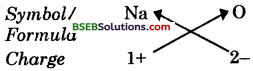Formula: Na6O

(ii) Formula of aluminium chloride.Formula: AlCl3

(iii) Formula of sodium sulphide .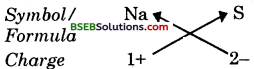Formula : Na2S

(iv) Formula of magnesium hydroxide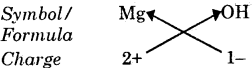Formula : Mg(OH)2

Question 2.
Write down the names of compounds represented by the following formulae :
(i) Al2(SO4)3
(ii) CaCl2
(iii) K2SO4
(iv) KNO3
(v) CaCSO3.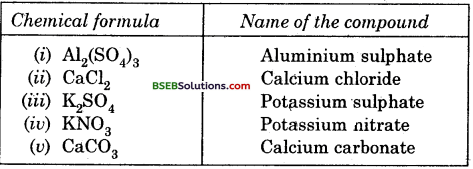Question 3.
What is meant by the term chemical formula ?
The chemical formula of a compound is a symbolic representation of its composition.Question 4.
How many atoms are present in a –
(i) H2S molecule and
(ii) PO42ion ?
(i) In H2S molecule there are three atoms. (Two atoms of hydrogen and one atom of sulphur).
(ii) In PO42ion there are five atoms. (One atom of phosphorous and four atoms of oxygen.)

Page 40

Question 1.
Calculate the molecular masses of H2, O2, Cl2, CO2, CH4, C2H6, C2H4, NH3, CH3OH.
(i) Molecular mass of H2
= 2 x Atomic mass of H
= 2 x 1u = 2 u

(ii) Molecular mass of O2
= 2 x Atomic mass of O
= 2 x 16M = 32 u

(iii) Molecular mass of Cl2
2 x Atomic mass of Cl = 2 x 35.5u = 71 u

(iv) Molecular mass of CO2 .
= Atomic mass of C + 2 x Atomic mass of O
= 12u x 2 x 16u = 12u + 32u = 44u

(v) Molecular mass of CH4
= Atomic mass of C + 4 x Atomic mass of H
= 12u x 4 x 1 u = 12u + 4u = 16u

(vi) Molecular mass of C2H6
= 2 x Atomic mass of C + 6 x Atomic mass of H
= 2 x 12u x 6 x lu = 24u + 6u = 30u(vii) Molecular mass of C2H4
= 2 x Atomic mass of C + 4 x Atomic mass of H
= 2 x 12u x 4 x 1 u = – 24u + 4u = 28u

(viii) Molecular mass NH3
= Atomic mass of N + 3 x Atomic mass of H
14u x 3 x 1u = 14u + 3u = 17u

(ix) Molecular mass of CH3OH
x = Atomic mass of C + 3 x Atomic mass of H + Atomic mass of O + Atomic mass, of H
= 12u + 3 x 1 u + 16u x 1u
= 12u + 3u + 16u + 1u = 32u

Question 2.
Calculate the formula unit masses of ZnO, Na2O, K2CO3, given atomic masses of Zn = 65 u, Na = 23 u, K = 39 u, C = 12 u, and O = 16 u.
(i) Atomic mass of Zn = 65 u
Atomic mass-of 0 = 16 u
Formula unit mass of ZnO
= At. mass of Zn + At. mass of O
= 65+16 = 81 u

(ii) Atomic mass of Na = 23 u
Atomic mass of 0 = 16 u
Formula unit mass of Na2O
= 2 x At. mass of Na + At. mass of O
= 2 x 23 + 16 = 46+16 = 62 u

(iii) Atomic mass of K = 39 u
Atomic mass of C = 12 u
Atomic mass of 0 = 16 u
Formula unit mass of K2CO3
= 2 x At. mass of K + At. mass of C + 3 x At. mass of O
= 2 x 39 + 12 + 3 x 16 = 78+12 + 48 = 138 uPage 42

Question 1.
If one mole of carbon atoms weighs 12 gram, what is the mass (in gram) of 1 atom of carbon ? “
Molar mass (M) of carbon atom = 12 g
Number (N) of particles (atoms) = 1
Avogadro’s number (No) = 6.023 x 1023
Given mass Number of particles x Molar mass Avogadro’s numberQuestion 2.
Which has more number of atoms, 100 grams of sodium or 100 grams of iron (given, atomic mass of Na = 23 u, Fe = 56 u ?
Given mass (m) of Na atoms = 100 g
Molar mass (M) of Na atoms = 23 g
Avogadro’s number (N0) = 6.023 x 1023
Number of particles (atoms)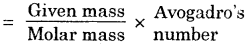Given mass (m) of Fe atoms = 1oo g
Molar mass (M) of Fe atoms = 56 g
Avogadro’s number (N0) = 6.023 x 1023
Number of particles (atoms)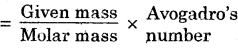⇒N = $$\frac {m}{M}$$ X N0
⇒N = $$\frac {100}{56}$$ x 6.023 x 1023
⇒N = 10.751 x 1023
Hence, 100 g of sodium contains more atoms.### Bihar Board Class 9 Science Chapter 3 Atoms and Molecules Textbook Questions and Answers

Question 1.
A 0.24 g sample of compound of oxygen and boron was found by analysis to contain 0.096 g of boron and 0.144 g of oxygen. Calculate the percentage composition of the compound by weight.
Percentage of BoronPercentage of Oxygen :Question 2.
When 3.00 g of carbon is burnt in 8.00 g oxygen, 11.00 g of carbon dioxide is produced. What mass of car bondioxide will be formed when 3.00 g of carbon is burnt in 50.00 g of oxygen? Which law of chemical combination will govern your answer ?
C + O2 → CO2Case I :
Mass of carbon = 3 g
Mass of oxygen = 8 g .
∴ Total mass of reactants = 3 + 8 = 11 g
Mass of product (CO2) = 11 g
Hence, total mass of reactants = Total mass of product

Case II :
Mass of carbon = 3 g
Mass of oxygen = 5 C
Total mass of reactants = 3 + 50 = 53 g
Using law of conservation of mass,
Total mass of reactants = Total mass of product Total mass of product (CO2) = 53 g

Question 3.
What are polyatomic ions ? Give examples.
Clusters of atoms act as an ion and these clusters are called polyatomic ions. They carry a fixed charge on them. For example, Ammonium (NH4)+, Sulphate (SO4)2-, Phosphate (PO4)3-etc.Question 4.
Write the chemical formulae of the following:
(a) Magnesium chloride
(b) Calcium oxide
(c) Copper nitrate
(d) Aluminium chloride
(e) Calcium carbonate.
(a) Formula of magnesium chloride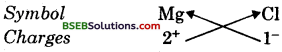∴ Formula of magnesium chloride = Mg1Cl2 or MgCl2

(b) Formula of calcium oxide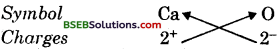∴ Formula of calcium oxide = Ca2O2 or CaO.

(c) Formula of copper nitrate∴ Formula of copper nitrate
⇒ Cu1(NO3)1 or CuNO3.

(d) For&ula of aluminium chloride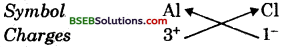∴ Formula of aluminium chloride = Al1Cl3orAlCl3.

(e) Formula of calcium carbonate∴ Formula of calcium carbonate = Ca2(CO3)2 or CaCO3Question 5.
Give the names of the elements present in  the following compounds.
(a) Quick lime
(b) Hydrogen bromide
(c) Baking powder
(d) Potassium sulphate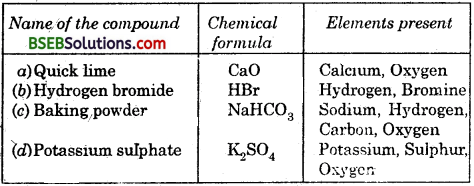Question 6.
Calculate the molar mass of the following substances:
(а) Ethyne, C2H2
(b) Sulphur molecule, S8
(c) Phosphorous molecule, P4 (Atomic mass of phosphorus = 31)
(d) Hydrochloric acid, HCl
(e) Nitric acid, HNO3
Mass of 1 mole molecule of a substance is called its molar mass.
(a) Atomic mass if carbon = 12 u
Atomic mass of Jiydrogen = 1 u
Molecular mass of C2H2
= 2 x At. mass of C + 2 x At. mass of H
= 2 x 12 + 2 x l = 24 + 2 = 26 u
∴ Molar mass of C2H2 = 26 g(b) Atomic mass of sulphur = 32 u
Molecular mass of S8 = 8 x 32 = 256 u
∴ Molar mass of S8 = 256 g

(c) Atomic mass of phosphorous = 31 u
Molecular mass of P2 = 4 x 31 = 124 u
∴ Molar mass of P4 = 124 g

(d) Atomic mass of hydrogen = 1 u
Atomic mass of chlorine = 35.5 u
Molecular mass of HCl
= At. mass of H + At. mass of Cl
= 1 + 35.5 = 36.5 u
Molar mass of HCl = 36.5 g

(e) Atomic mass of hydrogen = 1 u
Atomic mass of nitrogen = 14 u
Atomic mass of oxygen = 16 u
Molecular mass of HNO3
= At. mass of H + At. mass of N + 3 x At. mass – of O
= 1 + 14 + 3 x 16
= 1 + 14 + 48 = 63 u
Molar mass of HNO3 = 63 g

Question 7.
What is the mass of:
(a) 1 mole of nitrogen atoms?
(b) 4 moles of aluminium atoms (Atomic mass of aluminium =27)?
(c) 10 moles of sodium sulphite (Na2SO3)?
(a) Number (n) of moles = 1
Molar mass (M) of N atoms = 14g
Mass = Molar mass x Number of moles
⇒ m = M x n
⇒ m = 14 x 1 = 14 g(b) Number (n) of moles = 4
Molar mass (M) of aluminium ions = 27 g (Mass of an ion is same as that of mass of an atom of same element) .
Mass = Molar mass x Number of moles
⇒ m = M x n =4  rn = 27′ x 4 = 108 g

(c) Number (n) of moles = 10
Molar mass (M) of Na2SO3
= 2 x 23 + 32 + 3 x 16 + 46 + 32 + 48 = 126 g
Mass – Molar mass x Number of moles
⇒m = M x
⇒m = 126 x 10 = 1260 g

Question 8.
Convert into mole :
(а) 12 g of oxygen gas
(b) 20 g of water
(c) 22 g of carbon dioxide
(a) Atomic mass of oxygen gas = 32 u
Molar mass (M) of 02 = 32 g
Given mass (m) of oxygen = 12 g
Number of moles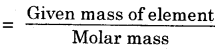n = $$\frac {m}{M}$$
n = $$\frac {12}{32}$$ = 0.375

(b) Molecular mass of water = 18 u
Molar mass (M) of water = 18 g
Given mass (m) of water = 20 g
Number of moles .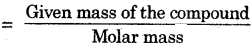n = $$\frac {m}{M}$$
n = $$\frac {20}{18}$$ = 1.11

(c) Molecular mass of carbon-dioxide = 44 u
Molar mass (M) of carbon-dioxide = 44 g
Given mas (m) of carbon-dioxide = 22 g
Number of moles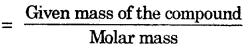n = $$\frac {m}{M}$$
n = $$\frac {22}{44}$$ = 0.5

Question 9.
What is the mass of:
(а) 0.2 mole of oxygen atoms ?
(b) 0.5 mole of water molecules ?
(a) Number (n) of moles= 0.2
Atomic mass of oxygen = 16 u
Molar mass (M) of oxygen atoms = 16 g
Mass = Molar mass x Number of moles
⇒ m = M x n
⇒ m = 16 x 0.2 = 3.2 g(b) Number (n) of moles = 0.5
Molecular mass of water = 18 u
Molar mass (M) of water = 18 g
Mass = Molar mass x Number of moles
⇒m = M x n
⇒ m = 18 x 0.5 = 9 g

Question 10.
Calculate the number of molecules of sulphur (Sg) present in 16 g of solid sulphur.
Atomic mass of sulphur = 32 u
Molar mass (M) of S8 molecule = 32 x 8 = 256 g
Given mass (m) of S8 molecule = 16 g
Avogadro’s number (N0) = 6.023 x 1023
Number (N) of particles (molecules)⇒ N = $$\frac {m}{M}$$ x N0
⇒ N = $$\frac {16}{256}$$ x 6.023 x 1023
⇒ N = 0.3764 x 1023 = 3.764 x 1022

Question 11.
Calculate the number of aluminium ions present in 0.051 g of aluminium oxide.
[Hint : The mass of an ion is the same as that of an atom of the same element. Atomic mass of A1 = 27u]
Molecular mass of aluminium oxide (Al0O3)
= 2.x 27 + 3×16 = 54 + 48 = 102 u
Molar mass (M) of Al2O3 = 102 g
Given mass (m) of Al2O3 = 0.051 g
Avogadro’s number (N0) = 6.023 x 1023
Number (N) of particles (ions)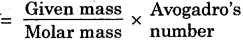⇒ N = $$\frac {m}{M}$$ x N0
⇒N = $$\frac {0.051}{102}$$ x 6.023 x 1023
⇒ N = 0.0030 x 1023
Since each molecule of Al2O3 contains two aluminium ions, so number of aluminium ions
= 2 x 0.0030 x 1023 = 0.006 x 1023 = 6 x 1020### Bihar Board Class 9 Science Chapter 3 Atoms and Molecules Textbook Activities

Activity 3.1

• Take one of the following sets, X and Y of chemicals

(i) copper sulphate sodium carbonate
(ii) barium chloride sodium sulphate

• Prepare separately a 5% solution of any one pair of substances listed under X and Y in water.
• Take a little amount of solution of Y in a conical flask and some solution of X in an ignition tube.
• Hang the ignition tube in the flask carefully; see that the solutions do not get mixed. Put a cork on the flask (see Fig. 3.1.)
• Weigh the flask with its contents carefully.• Now tilt and swirl the flask, such that the solutions X and Y get mixed.
• Weigh again.

Question 2.
(a) What happens in the reaction flask ?
(b) Do you think that a chemical reaction has taken place ?
(c) Why should we put a cork on the mouth of the flask ?
(d) Does the mass of the flask and its contents change ?
(a) In the first case, the chemicals do not mix with each other but in the second case, when the flask is tilted and swirled, the chemicals get mixed.
(b) In the first case, no chemical reaction takes place. In the second case, depending upon the chemicals chosen, the following reactions take place :(c) We put a cork on the mouth of the flask so that ah’ from outside does not enter the flask. Air may react with the chemicals present in the ignition tube or the conical flask.
(d) No, the mass of the flask does not change. It verifies the law of conservation of mass.Activity 3.2

Table 3.1. Molecules of some compounds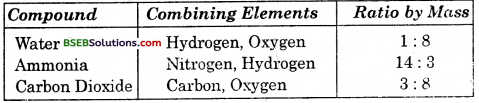Table 3.2. Atomic masses of a few elements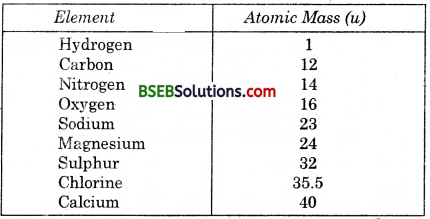Refer to Table 3.1 for ratio by mass of atoms present in molecules and Table 3.2 for atomic masses of elements. Find the ratio by number of the atoms of elements in the molecules of compounds given in Table 3.1.
(i) The ratio by number of atoms for a water molecule can be found as follows :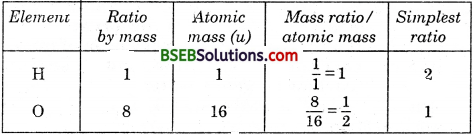Thus, the ratio by number of atoms for water H : O = 2 : 1.

(ii) The ratio by number of atoms for an molecule can be found as follows :Thus, the ratio by number of atoms for ammonia is N : H = 1 : 3.

(iii) The ratio by number of atoms for a carbon-dioxide molecule can be found as follows :### Bihar Board Class 9 Science Chapter 3 Atoms and Molecules Additional Important Questions and Answers

Question 1.
In which year was the idea of divisibility of matter considered in India ?
The idea bf divisibility of matter was considered in India around 500 B.C.

Question 2.
Which philosophers had always wondered about the unknown and unseen form of * matter ? •
Ancient Indian and Greek philosophers had always wondered about the unknown and unseen form of matter.

Question 3.
Name two Indian philosophers who studied about the particles of matter.

Question 4.
What name was given to the smallest particles of matter by Maharishi Kanad ?
The smallest particles of matter were named “Parmanu” by Maharishi Kanad.

Question 5.
During which century did the scientists recognize the difference between elements and compounds ?
By the end of the eighteenth century, scientists recognized the difference between elements and compounds. ,

Question 1.
Write the postulate given by the Indian philosopher Maharishi ,Kanad.
Indian philosopher, Maharishi Kanad postulated that if we go on dividing matter (padarth), we shall get smaller and smaller particles and a limit will come when we will come across smallest particles beyond which further division will not be possible. ,

Question 2.
What was the Indian philosopher, Pakudha Katyayama’s contribution.to Maharishi Eanad’s postulate ?
Pakudha Katyayama elaborated Kanad’s .. doctrine and said that these particles normally exist in a combined form, which gives us various forms of matter.

Question 3.
What was the suggestion given by the Greek philosophers Democritus and Leucippus regarding matter ?
The Greek philosophers Democritus and Leucippus suggested that if we go on dividing matter, a stage will come when particles obtained cannot be divided further. They called these indivisible particles atom (a-tomio—indivisible).

Question 4.
Define a molecule.
The molecule can be defined as the smallest particle of an element or a compound that is capable of an independent existence and shows all the properties of that substance. Atoms of same or different elements can join together to form molecules.

Question 1.
Write the rules for writing symbols. Give an example of each rule.